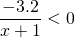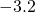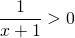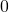# Solution assignment 04 Inequalities with fractions

### Assignment 4

Solve:### Solution

It is less easy to work with negative numbers and therefore we divide left- and right-hand side by. Then we get (note that dividing by a negative number changes the direction of the inequality sign) :The numerator is positive for all values ofand thus the fraction will be positive if also the denominator will be greater than, so if:0